# Completed Square Form

The completed square form can be used to:

1. Locate the vertex of a parabola, bothandcoordinates;
3. Calculate the inverse of a quadratic function – discussed in the grade 12 curriculum.

# A Perfect Squareis known as a perfect square because it can be expressed as a single term squared:We can confirm this by multiplying out the brackets on the right hand side:is almost a perfect square. It is just one unit more than. It can be written:‘Completing the square’ is to assume that your quadratic expression is a perfect square, and then to make an adjustment so that the constant term is correct.

Remember that in general, a perfect square has the form. (Assuming.)

Suppose the following are perfect squares – what is the constant?

1.2.3.# Completing the Square whenWhen the coefficient of thetermwe can write the quadratic expressionin completed square form using this process:

• half the coefficient, and write;
• subtract the square of, to write;
• Add the original constant and simplify,.

For example:• half the coefficient, write. This is equal to;
• subtract the square of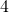: writeThis is equal to;
• Add the original constant and simpilfy: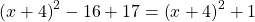This is equal to.

These three steps can all be done in one line: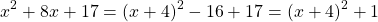## Example 1Half ofis;.For brain muscle memory: ‘half the coefficient, subtract its square, remember original constant’.

## Example 2Half of, is, the square ofis.To check that the right hand side is equal to the left hand side, lets multiply out and simplify:## Example 3

‘half the coefficient of,  subtract its square’## Example 4, odd coefficient of# Practice

Use this applet to develop muscle memory for the complete the square process:

# When the coefficient ofis not 1

How we complete the square depends if we are solving an equation or rewriting an expression.

If we have an equation, we can divide both sides by the coefficient:(remember that). The rest of this work is detailed in Example 4 on another page.

If we have an expression, we need to factor the coefficient of:We can then complete the square within the large brackets: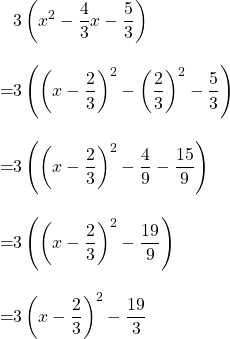This algebra is fairly tricksome and can take some practice to master. However, in a pinch, one might wish to use the following:

# Using the Vertex to Complete the Square

On another page, we argue that if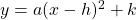, the vertex has coordinates. We can find the valuesandusing any ‘find the vertex’ method.

On a different page, we see that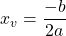Considering our example above,,. Therefore,.

To findwe simply need to substituteto the expression:Therefore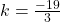.

After calculatingand, (), we havewhich agrees with the algebra above.